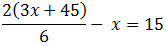No. of Players: 2+ Type of Game: written What you need: pen and paper

## Goal

To make the highest-valued three-letter word.

## How to play

Letters are given point values according to their relative position in the alphabet. So A = 1 point, B = 2, C = 3, and so on, to Z = 26. The first player writes down a three-letter word and adds up its point total. The second player writes down another three-letter word that starts with one of the letters in the first word, and adds up its point total. This point total must be higher than the point total of the first word. The next player does the same, and so on. Once a player cannot make a word with a higher point total than the previous word, the game ends. The player who made the word with the highest point total is the winner. No word can be used twice.

Another variation of this game is One Hundred.

## Example

The players agree to kick-off the game by selecting a three-letter word at random. They do this to remove the first player’s advantage in setting the pace of the game. This is because words formed using letters from the beginning of the alphabet are valued much lower than those using letters from the end of the alphabet. So they open a book to a random page and select the first three-letter word they find. That word is CAP.

Andrea writes down CAP which has a point total of (3+1+16) = 20.
Beth writes down PAT = (16+1+20) = 37.
Clark writes down TOP = (20+15+16) = 51.
Daisy writes down TOY = (20+15+25) = 60.
Andrea writes down TUX = (20+21+24) = 65.

After some debate, the players find Andrea's last word acceptable. And since no other player can think of a word with a higher point total, she wins the game.

Did you know?

At your next get-together with friends or colleagues, you can convincingly pass yourself off as a mathematical genius. Here's how to do it.

Math Trick #1

First, select someone. Tell them to think of any number – no matter the number of digits. Advise them that if they have a large or complex number, they might need a calculator for what comes next. (Having one handy to give them is a nice touch.) Then tell them to:

Multiply the number by 3.

Double it.

Divide by 6.

Subtract the original number.

Then (taking your time for dramatic effect, of course) you announce the answer is 15. And they are uttery amazed that the answer is actually 15!

But what you don't tell them is that the answer is always 15 for any number you start with, as long as the mathematical operations above are exactly followed each and every time. So be sure not to repeat this trick immediately with the same people, or they will surely spot the pattern. Or else you'll need another trick up your sleeve.

For math lovers,
here's the

equationMath Trick #2

Again, select someone and tell them to think of any number. Then have them:

Multiply it by 3.

Divide by 3.

Subtract the original number.

Then step back and hold up 2 fingers. Because the answer (everytime with these steps) is 2!

That's all it takes. Just memorize the steps and answers to these two simple tricks, and you'll be a math superstar at your next party!

Here's the

equationHome